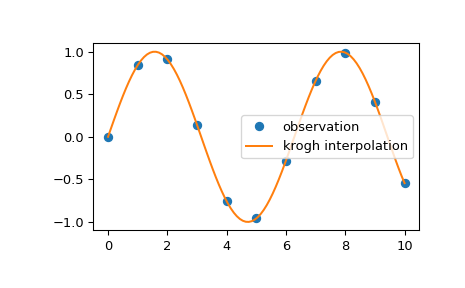# scipy.interpolate.krogh_interpolate¶

scipy.interpolate.krogh_interpolate(xi, yi, x, der=0, axis=0)[source]

Convenience function for polynomial interpolation.

See KroghInterpolator for more details.

Parameters
xiarray_like

Known x-coordinates.

yiarray_like

Known y-coordinates, of shape (xi.size, R). Interpreted as vectors of length R, or scalars if R=1.

xarray_like

Point or points at which to evaluate the derivatives.

derint or list, optional

How many derivatives to extract; None for all potentially nonzero derivatives (that is a number equal to the number of points), or a list of derivatives to extract. This number includes the function value as 0th derivative.

axisint, optional

Axis in the yi array corresponding to the x-coordinate values.

Returns
dndarray

If the interpolator’s values are R-D then the returned array will be the number of derivatives by N by R. If x is a scalar, the middle dimension will be dropped; if the yi are scalars then the last dimension will be dropped.

KroghInterpolator

Krogh interpolator

Notes

Construction of the interpolating polynomial is a relatively expensive process. If you want to evaluate it repeatedly consider using the class KroghInterpolator (which is what this function uses).

Examples

We can interpolate 2D observed data using krogh interpolation:

>>> import matplotlib.pyplot as plt
>>> from scipy.interpolate import krogh_interpolate
>>> x_observed = np.linspace(0.0, 10.0, 11)
>>> y_observed = np.sin(x_observed)
>>> x = np.linspace(min(x_observed), max(x_observed), num=100)
>>> y = krogh_interpolate(x_observed, y_observed, x)
>>> plt.plot(x_observed, y_observed, "o", label="observation")
>>> plt.plot(x, y, label="krogh interpolation")
>>> plt.legend()
>>> plt.show()#### Previous topic

scipy.interpolate.barycentric_interpolate

#### Next topic

scipy.interpolate.pchip_interpolate